### Practice Set 6.1 Trigonometry Class 10th Mathematics Part 2 MHB Solution

Practice Set 6.1

1. If sintegrate heta = 7/25 find the values of cosθ and tanθ.
2. sin^2theta /costheta +costheta = sectheta Prove that:
3. If tantheta = 3/4 find the values of secθ and cosθ.
4. If cottheta = 40/9 find the values of cosecθ and sinθ.
5. If 5secθ- 12cosecθ = 0, find the values of secθ, cosθ and sinθ.
6. If tanθ = 1 then, find the values of sintegrate heta +costheta /sectheta +cosectheta…
7. cos^2theta (1+tan^2theta) = 1 Prove that:
8. root 1-sintegrate heta /1+sintegrate heta = sectheta -tantheta Prove that:…
9. (sec θ - cos θ) (cot θ + tan θ) = tan θ sec θ Prove that:
10. cot θ + tan θ = cosec θ sec θ Prove that:
11. 1/sectheta -tantheta = sectheta +tantheta Prove that:
12. sin^4 θ - cos^4 θ = 1 - 2cos^2 θ Prove that:
13. sectheta +tantheta = costheta /1-sintegrate heta Prove that:
14. If tantheta + 1/tantheta = 2 then show that tan^2theta + 1/tan^2theta = 2 Prove that:…
15. tana/(1+tan^2a)^2 + cota/(1+cot^2a)^2 = sinacosa Prove that:
16. sec^4 A (1- sin^4 A) - 2tan^2 A = 1 Prove that:
17. tantheta /sectheta -1 = tantheta +sectheta +1/tantheta +sectheta -1 Prove that:…

###### Practice Set 6.1

We know that,

sin2θ + cos2θ = 1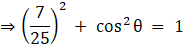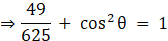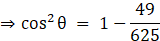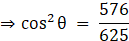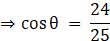Also,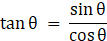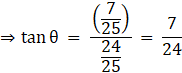Question 2.

Prove that: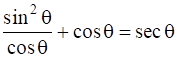Taking LHS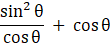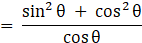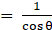[As, sin2θ + cos2θ = 1]

= sec θ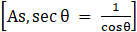= RHS

Proved !

Question 3.

If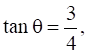find the values of secθ and cosθ.

We know that,

sec2θ= 1 + tan2θ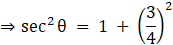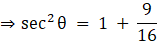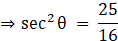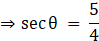Also,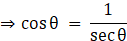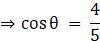Question 4.

If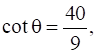find the values of cosecθ and sinθ.

We know that,

cosec2θ = 1 + cot2θ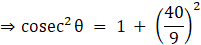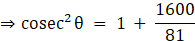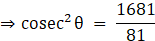⇒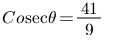Also,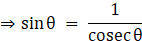∴ SinΘ=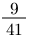Question 5.

If 5secθ- 12cosecθ = 0, find the values of secθ, cosθ and sinθ.

5secθ - 12cosecθ = 0

⇒ 5secθ = 12cosecθ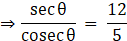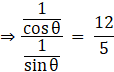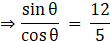As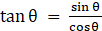we have,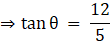Also, We know that,

sec2θ= 1 + tan2θ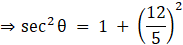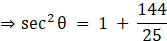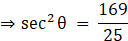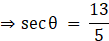Also,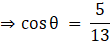Now, again using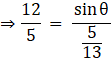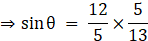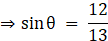Question 6.

If tanθ = 1 then, find the values of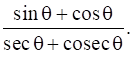Given,

tan θ = 1

⇒ θ = 45° [as tan 45° = 1]

Also,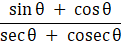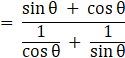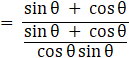= sinθ cosθ

= sin 45° cos 45°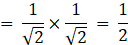Question 7.

Prove that: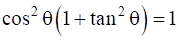Taking LHS

cos2θ(1 + tan2θ)

= cos2θ sec2θ [As, sec2θ = 1 + tan2θ]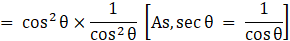= 1

= RHS

Proved !

Question 8.

Prove that: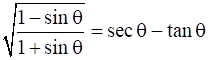Taking LHS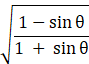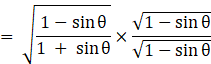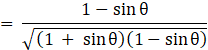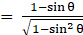[(a + b)(a - b) = a2 - b2 ]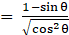[As, sin2θ + cos2θ = 1]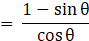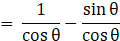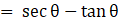= RHS

Proved !

Question 9.

Prove that:

(sec θ – cos θ) (cot θ + tan θ) = tan θ sec θ

Taking LHS

(secθ - cosθ)(cotθ + tanθ)

= secθcotθ + secθtanθ - cosθcotθ - cosθtanθ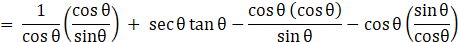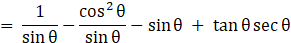Taking LCM of first three terms,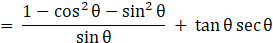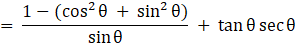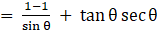[As, sin2θ + cos2θ = 1]

= tanθsecθ

= RHS

Proved !

Question 10.

Prove that:

cot θ + tan θ = cosec θ sec θ

Taking LHS, and putting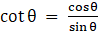and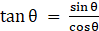= cotθ + tanθ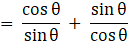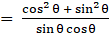[As, sin2θ + cos2θ = 1]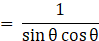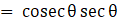= RHS

Proved !

Question 11.

Prove that: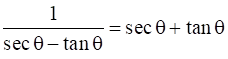Taking LHS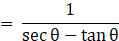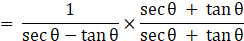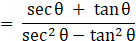= secθ + tanθ [As, sec2θ = 1 + tan2θ ⇒ sec2θ - tan2θ = 1]

= RHS

Proved !

Question 12.

Prove that:

sin4 θ – cos4 θ = 1 – 2cos2 θ

L.H.S = sin4θ – cos4θ

= (sin2θ – cos2θ)(sin2θ + cos2θ)

= (sin2θ – cos2θ)

= (1 – cos2θ – cos2θ)

= 1- 2cos2θ

Question 13.

Prove that: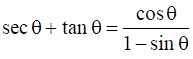Taking RHS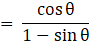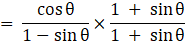(Multiplying both Numerator and Denominator by 1+SinΘ)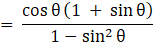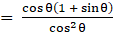[As, sin2θ + cos2θ = 1]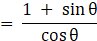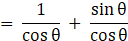= secθ + tanθ

= LHS

Proved !

Question 14.

Prove that:

If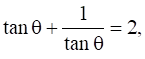then show that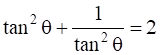Given,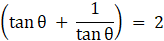Squaring both side,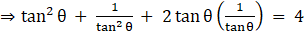[(a + b)2 = a2 + b2 + 2ab]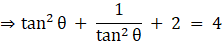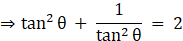Hence, Proved !

Question 15.

Prove that: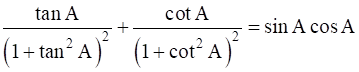Taking RHS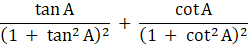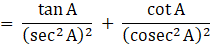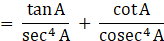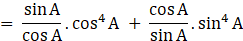= sinA cos3A + cosA sin3A

= sinAcosA(cos2A + sin2A) [As, sin2θ + cos2θ = 1]

= sinAcosA

= RHS

Proved !

Question 16.

Prove that:

sec4A (1– sin4A) – 2tan2 A = 1

Taking LHS

= sec4A(1 - sin4A) - 2tan2A

= sec4A - sin4A sec4A - 2tan2A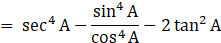= sec4A - tan4A - tan2A - tan2A

= sec4A - tan2A(1 + tan2A) - tan2A

= sec4A - tan2A sec2A - tan2A [As, sec2θ = 1 + tan2θ ]

= sec2A(sec2A - tan2A) - tan2A

= sec2A - tan2A [As, sec2θ = 1 + tan2θ ⇒ sec2θ - tan2θ = 1]

= 1

= RHS

Proved !

Question 17.

Prove that: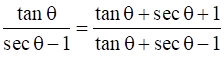Taking RHS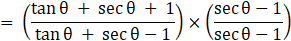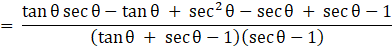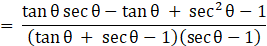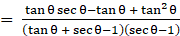[As sec2θ -1 = tan2θ ]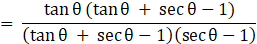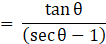= LHS

Proved.

## PDF FILE TO YOUR EMAIL IMMEDIATELY PURCHASE NOTES & PAPER SOLUTION. @ Rs. 50/- each (GST extra)

SUBJECTS

HINDI ENTIRE PAPER SOLUTION

MARATHI PAPER SOLUTION
SSC MATHS I PAPER SOLUTION
SSC MATHS II PAPER SOLUTION
SSC SCIENCE I PAPER SOLUTION
SSC SCIENCE II PAPER SOLUTION
SSC ENGLISH PAPER SOLUTION
SSC & HSC ENGLISH WRITING SKILL
HSC ACCOUNTS NOTES
HSC OCM NOTES
HSC ECONOMICS NOTES
HSC SECRETARIAL PRACTICE NOTES

2019 Board Paper Solution

HSC ENGLISH SET A 2019 21st February, 2019

HSC ENGLISH SET B 2019 21st February, 2019

HSC ENGLISH SET C 2019 21st February, 2019

HSC ENGLISH SET D 2019 21st February, 2019

SECRETARIAL PRACTICE (S.P) 2019 25th February, 2019

HSC XII PHYSICS 2019 25th February, 2019

CHEMISTRY XII HSC SOLUTION 27th, February, 2019

OCM PAPER SOLUTION 2019 27th, February, 2019

HSC MATHS PAPER SOLUTION COMMERCE, 2nd March, 2019

HSC MATHS PAPER SOLUTION SCIENCE 2nd, March, 2019

SSC ENGLISH STD 10 5TH MARCH, 2019.

HSC XII ACCOUNTS 2019 6th March, 2019

HSC XII BIOLOGY 2019 6TH March, 2019

HSC XII ECONOMICS 9Th March 2019

SSC Maths I March 2019 Solution 10th Standard11th, March, 2019

SSC MATHS II MARCH 2019 SOLUTION 10TH STD.13th March, 2019

SSC SCIENCE I MARCH 2019 SOLUTION 10TH STD. 15th March, 2019.

SSC SCIENCE II MARCH 2019 SOLUTION 10TH STD. 18th March, 2019.

SSC SOCIAL SCIENCE I MARCH 2019 SOLUTION20th March, 2019

SSC SOCIAL SCIENCE II MARCH 2019 SOLUTION, 22nd March, 2019

XII CBSE - BOARD - MARCH - 2019 ENGLISH - QP + SOLUTIONS, 2nd March, 2019

HSC Maharashtra Board Papers 2020

(Std 12th English Medium)

HSC ECONOMICS MARCH 2020

HSC OCM MARCH 2020

HSC ACCOUNTS MARCH 2020

HSC S.P. MARCH 2020

HSC ENGLISH MARCH 2020

HSC HINDI MARCH 2020

HSC MARATHI MARCH 2020

HSC MATHS MARCH 2020

SSC Maharashtra Board Papers 2020

(Std 10th English Medium)

English MARCH 2020

HindI MARCH 2020

Hindi (Composite) MARCH 2020

Marathi MARCH 2020

Mathematics (Paper 1) MARCH 2020

Mathematics (Paper 2) MARCH 2020

Sanskrit MARCH 2020

Important-formula

THANKS